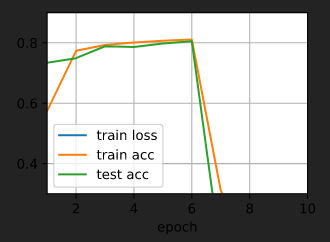# Implementation of Multilayer Perceptrons

1.When test accuaracy increases most quickly and high, can we say that this hyperparameter is the best value?

Unless you are sure the given optimization function is convex, we hardly ever say the “best” model or “best” set of hyperparameters.

``````def net(X):
X = X.reshape((-1, num_inputs))
H = relu(X@W1 + b1)   # Here '@' stands for dot product operation
return (H@W2 + b2)
``````

In the last line shouldn’t we have applied the softmax function to the return value `H@W2 + b2`? Isn’t there a chance that this operation would yield a negative value or a value greater than 1?

When I do use the softmax function, the train loss dissapears and the accuracy suddenly drops to 0. What could be the cause behind this?In the last line shouldn’t we have applied the softmax function to the return value `H@W2 + b2` ? Isn’t there a chance that this operation would yield a negative value or a value greater than 1?

We use the loss function to process the output values of `net(X)`, so it does not need to yield a value in (0, 1).

When I do use the softmax function, the train loss dissapears and the accuracy suddenly drops to 0. What could be the cause behind this?

Could you show us the code so that we can reproduce the results?

Hey,

The `CrossEntropyLoss` function already computes the Softmax.

1 Like

Oh I see. Thanks @manuel-arno-korfmann

What’s your IDE? I’m curious. Thanks.

I think it’s vscode with plugins about viewing notebook

@Xiaomut
I am using vscode, and I can’t find this.
@Kushagra_Chaturvedy

how do you use the softmax during testing? since softmax is implemented in loss function, the output of net(x) doesn’t apply softmax to its output. and the result of the argmax is the max of logits not the probability. I wonder how do you use softmax when testing

@ccpvirus
We use softmax to calculate probablility first, and then find the max probabillity one.

Hi @ccpvirus, as @StevenJokes mentioned we use the “maximum” value across the 10 class outputs as our final label. As softmax is just a “rescaling” function, it doesn’t affect whether a prediction output (i.e., a class lable) is or isn’t the maximum over all classes. Let me know whether this is clear to you.2 Likes

Dear all, may I know why we use torch.randn to initialize the parameter here instead of using torch.normal as in Softmax Regression implementation? Are there any advantages? Or actually there are no big differences, we can use both of them? Thanks.

W1 = nn.Parameter(torch.randn(

@Gavin
For a standard normal distribution (i.e. `mean=0` and `variance=1` ), you can use `torch.randn`
For your case of custom `mean` and `std` , you can use torch.normal

2 Likes

Hello. Can you please advise why 0.01 is being multiplied after generating the random numbers?
W1 = nn.Parameter(torch.randn(
Thanks,i am starting it